# How to use SolverJS ?

• Last Updated : 10 Sep, 2021

SolverJS is a JavaScript library, it contains a lot of mathematical and basic logical function which is used very frequently in any application. It aims at solving logical problems easily with a simple call to certain functions also all functions are time and space-efficient. It has a variety of use-cases, for example, conversion-related, matrix operations, basic string operations, and mathematical utilities like gcd, power, prime checking, etc. In this article, we are going to discuss the approach to use this library and later some problem statements in which we can use it.

Let’s see step-by-step implementation to get an idea about how to use this library and at the end of the article you will be pretty comfortable with it to use in your projects.

Hey geek! The constant emerging technologies in the world of web development always keeps the excitement for this subject through the roof. But before you tackle the big projects, we suggest you start by learning the basics. Kickstart your web development journey by learning JS concepts with our JavaScript Course. Now at it's lowest price ever!

Step 1: Create a Node application. As we are going to work with a library that works on node.js hence it is compulsory to create a node project first, So type this command in your terminal to create a node application.

Syntax:

`npm init `

This command will ask for few configurations about the project you can very easily fill them, also if you’ve mistakenly press enter for some field or if you want to change something, you always have an option to change these configurations later from the package.json file.

Step 2: Installation of the library using npm. Run this command in your terminal to install the library using the node package manager, if you’re using yarn package manager then use `yarn add` instead of `npm install`.

Syntax:

`npm install solverjs `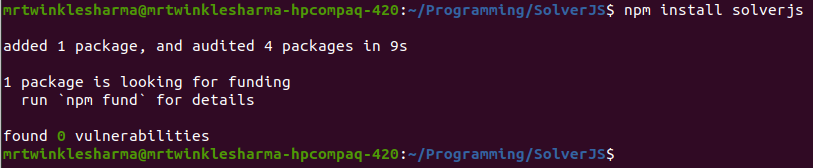Step 3: Import in your application file. Create a javascript file, let’s name it app.js, and Import the library in your code before using it. Below the syntax is given to import a library in a node application. We provide the name of the library inside the parenthesis of require keyword and then store the result in a const variable.

`const solverjs = require('solverjs');`

Project Structure: This will be our folder structure, In the entire article, we will work with this app.js.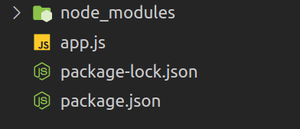Step 4:  Call relevant function and Use. Now we will create one function and start using the library in our project.

Note: After the step 1 and 2, you can call any function available in the library with providing required arguments and that function will return the appropriate result.

For example, if you want to calculate the average of an array then simply call the function avg([array]) and it will return the average.

## app.js

 `//Import the library``const solverjs = require(``'solverjs'``);`` ` `const myArray = [5,2,1,8,4];`` ` `//Call function``const calculatedAverage = solverjs.avg(myArray);``console.log(calculatedAverage);`

Step to run the application: Open the terminal and type the following command.

`node app.js`

Output: This is the output of the above code.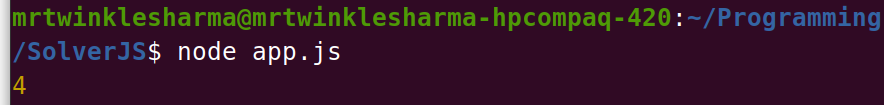Now let’s see the area of usage where we can use this library with the help of examples.

1. Conversions: The library contains many functions related to the conversion from one physical unit to another, There are various situations where our application needs these conversions, So we can achieve our goal just in a simplistic function call. The basic structure of every function in this category looks like this:

```Name: someUnitToAnotherRequiredUnit()
Argument: valueInGivenUnit
Return Value: valueInRequiredUnit ```

1.1 Length-related conversions:

• Example 1: Here we are converting yards to meter, function ydToMe() receives length in yards and returns the length in meter. After getting the result we are simply printing it with console.log and template literals.
Note:- In case you don’t know about template literals, let me tell you that it is used to generate a dynamic string in ES6 and you can inject a javascript expression inside \${}.

## app.js

 `const solverjs = require(``'solverjs'``);`` ` `const lengthInYard = 5;``const lengthInMetre = solverjs.ydToMe(lengthInYard);``console.log(`\${lengthInYard} yd = \${lengthInMetre} m`);`

Output: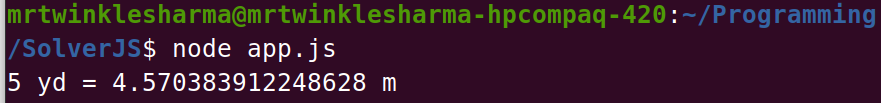• Example 2: Here we are converting feet to centimeters, function ftToCm() receives length in feet and returns the length in centimeters.

## app.js

 `const solverjs = require(``'solverjs'``);`` ` `const lengthInFeet = 2;``const lengthInCentimetre = solverjs.ftToCm(lengthInFeet);``console.log(`\${lengthInFeet} ft = \${lengthInCentimetre} cm`);`

Output: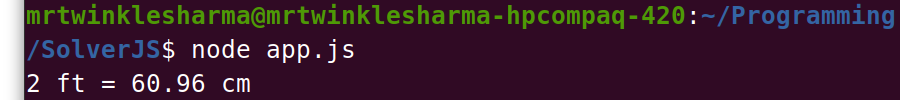1.2 Area-related conversions:

• Example 1: Here we are converting square meters to square inches, function sqMeToSqIn() receives area in square meters and returns the area in square inches.

## app.js

 `const solverjs = require(``'solverjs'``);`` ` `const areaInSquareMetre  = 25;``const areaInSquareInch = solverjs.sqMeToSqIn(areaInSquareMetre);``console.log(`\${areaInSquareMetre} sq. m. = \${areaInSquareInch} sq. ``in``.`);`

Output: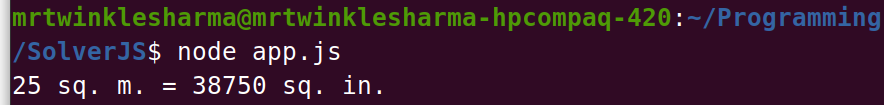• Example 2: Here we are converting hectares to square kilometers, function hectToSqKm() receives area in hectares and returns the area in square kilometers.

## app.js

 `const solverjs = require(``'solverjs'``);`` ` `const areaInHectare = 2;``const areaInSquareKilometre = solverjs.hectToSqKm(areaInHectare);``console.log(`\${areaInHectare} hect = \${areaInSquareKilometre} sq. km.`);`

Output: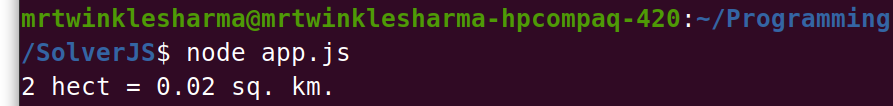1.3 Digital Storage-related conversions:

• Example 1: Here we are converting bytes to bits, function byteToBit() receives memory in bytes and returns the memory in bits.

## app.js

 `const solverjs = require(``'solverjs'``);`` ` `const storageInByte = 600;``const storageInBit  = solverjs.byteToBit(storageInByte);``console.log(`\${storageInByte} byte = \${storageInBit} bit`);`

Output: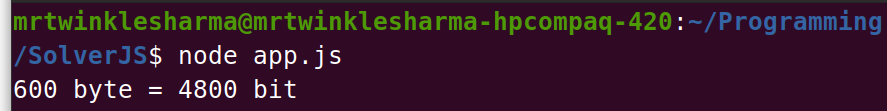• Example 2: Here we are converting GIgabytes to Kilobytes, function gbToKb() receives memory in GIgabytes and returns the memory in Kilobytes.

## app.js

 `const solverjs = require(``'solverjs'``);`` ` `const storageInGigaByte = 1.5;``const storageInKiloByte  = solverjs.gbToKb(storageInGigaByte);``console.log(`\${storageInGigaByte} gb = \${storageInKiloByte} kb`);`

Output: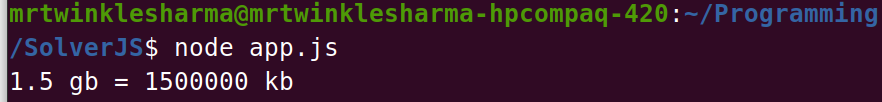1.4 Time-related Conversion:

• Example 1: Here we are converting Nanoseconds to Microseconds, function nsToUs() receives time in Nanoseconds and returns time in Microseconds.

## app.js

 `const solverjs = require(``'solverjs'``);`` ` `const timeInNanoSecond = 1500;``const timeInMicroSecond  = solverjs.nsToUs(timeInNanoSecond);``console.log(`\${timeInNanoSecond} ns = \${timeInMicroSecond} microsecond`);`

Output: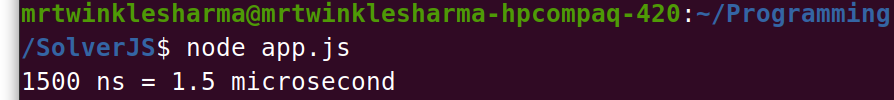• Example 2: Here we are converting Nanoseconds to Months, function nsToMm() receives time in Nanoseconds and returns time in months.

## app.js

 `const solverjs = require(``'solverjs'``);`` ` `const timeInNanoSecond = 200000000;``const timeInMonth  = solverjs.nsToMm(timeInNanoSecond);``console.log(`\${timeInNanoSecond} ns = \${timeInMonth} month`);`

Output: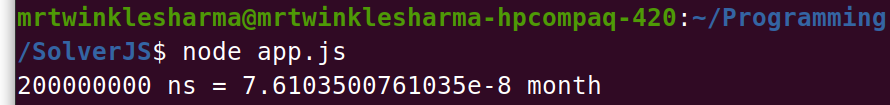1.5 Temperature-related conversions:

• Example 1: Here we are converting celsius to kelvin, function celToKel() receives temperature in celsius and returns the temperature in kelvin.

## app.js

 `const solverjs = require(``'solverjs'``);`` ` `const temperatureInCelsius = 4;``const temperatureInKelvin  = solverjs.celToKel(temperatureInCelsius);``console.log(`\${temperatureInCelsius} celsius = \${temperatureInKelvin} kelvin`);`

Output: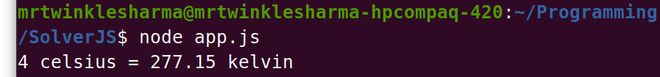• Example 2: Here we are converting Kelvin to Fahrenheit, function kelToFah() receives temperature in Kelvin and returns the temperature in Fahrenheit.

## app.js

 `const solverjs = require(``'solverjs'``);`` ` `const temperatureInKelvin = 500;``const temperatureInFahreinheit  = solverjs.kelToFah(temperatureInKelvin);``console.log(`\${temperatureInKelvin} kelvin = \${temperatureInFahreinheit} fahreinheit`);`

Output: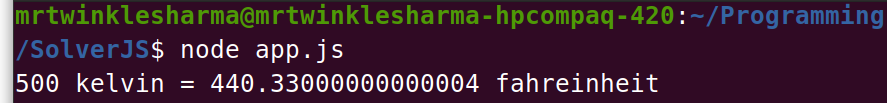There are many conversion methods available in this library, which are not used here but, the syntax, the way we use them, and the working remain the same so you can explore those on your own very easily.

2. Number System Conversions: There are many functions related to the conversion of numbers from one number system to another. The basic structure of every function in this category looks like this:

```Name: numberInOneNumberSystemToNumberInAnotherNumberSystem()
Argument: numberInGivenNumberSystem
Return Value: numberInRequiredNumberSystem ```

Below are the two examples of conversion among different number systems.

Example 1: Here we are converting a hexadecimal number to octal, function hexToOct() receives number in hexadecimal and returns the number in octal.

## app.js

 `const solverjs = require(``'solverjs'``);`` ` `const hexNumber  = ``'12A'``;``const octNumber = solverjs.hexToOct(hexNumber);``console.log(`\${hexNumber} ``in` `hexadecimal  = 0o\${octNumber} ``in` `octal`);`

Output: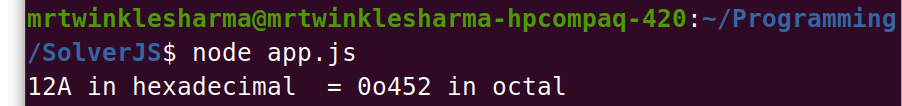Example 2: Here we are converting a decimal number to binary, function decToBin() receives the number in the decimal number system and returns the number in the binary number system.

## app.js

 `const solverjs = require(``'solverjs'``);`` ` `const decimalNumber  = 25;``const binaryNumber = solverjs.decToBin(decimalNumber);``console.log(`\${decimalNumber} ``in` `decimal = \${binaryNumber} ``in` `binary`);`

Output: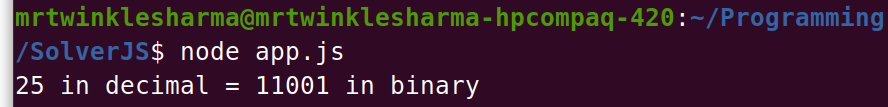3.Matrix Operations: Matrix operations are very common in any application this library provides various functions to manipulate matrix. two common are practiced below, addition and subtraction of matrices.

Example: At the starting of the program we are creating two matrices to perform operations,
The matAdd() function receives two matrices as arguments and returns the addition of those matrices, we have stored the result in a variable. The matSub() function receives two matrices as arguments and returns the subtraction of second by first of those matrices, we have also stored the result in another variable. At the end of the program, we are simply printing those created variables, actually, they are 2-dimensional arrays so we can run a for loop to print the whole array.

## app.js

 `const solverjs = require(``'solverjs'``);``const matrix1 = [ ``        ``[1,2,4],``        ``[6,3,9],``        ``[9,5,5]``    ``];``const matrix2 = [ ``    ``[3,1,9],``    ``[10,12,2],``    ``[19,8,5]``];`` ` `const matrixSum12 = solverjs.matAdd(matrix1, matrix2);``const matrixSubtract12 = solverjs.matSub(matrix1, matrix2);`` ` `console.log(``"Sum of Given Matrices"``);``for``(let i = 0;i<3;i++) console.log(matrixSum12[i]);   `` ` `console.log(``"Subtraction of 2nd matrix from 1st"``);``for``(let i = 0;i<3;i++) console.log(matrixSubtract12[i]);`

Output: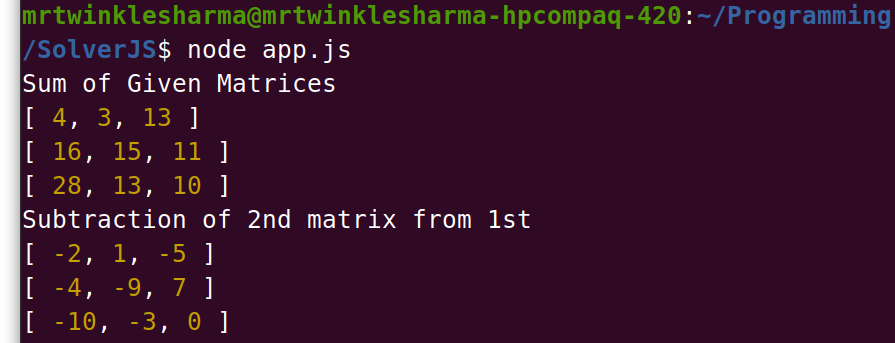4. Mathematical Logics: Mathematics is everywhere in programming, we need it frequently solverJS provides a lot of functions below are the 5 examples,

Example 1: Here we are finding whether a number is a palindrome or not, the function isPalindrome(), receives a number, and returns true or false based on the condition that the number is a palindrome or not. We are using the ternary operator to simply execute a piece of code on true or other on false. In our case, the 1st console.log statement will be executed.

## app.js

 `const solverjs = require(``'solverjs'``);`` ` `const num1  = 12233221;``solverjs.isPalindrome(num1) ? console.log(`\${num1} is Palindrome`) :``console.log(`\${num1} is not Palindrome`);`

Output: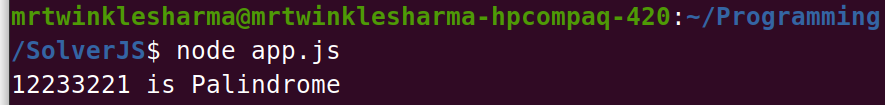Example 2: Here we are finding a number that is reverse to the given number, the function reverse(), receives a number, and returns the reverse number according to the given number.

## app.js

 `const solverjs = require(``'solverjs'``);`` ` `const num2 = 43215; ``const num2Reverse = solverjs.reverse(num2);``console.log(`Reverse of \${num2} is \${num2Reverse}`);`

Output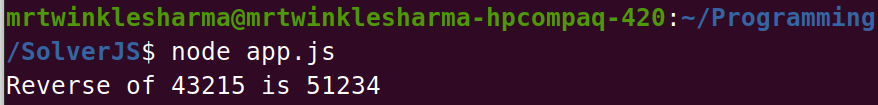Example 3: Here we are finding the factorial of the given number, fac() this function a receives number and returns the factorial.

## app.js

 `const solverjs = require(``'solverjs'``);`` ` `const num6 = 8;``const num6Factorial = solverjs.fac(num6);``console.log(`Factorial of \${num6} is \${num6Factorial}`);`

Output: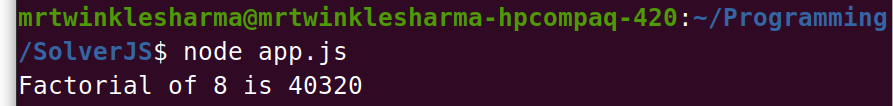Also, the library provides much more methods that are not used here, like power function, printing Fibonacci series, finding Fibonacci, calculating mod, etc.

5. String Operations: These are some of the functions related to operations on a string, in 1st example, we are finding total words in a given sentence, and in the second we are finding total permutations on a given word.

Example 1: Here we are finding the number of words in a given string, wordCount() function receives a string and a separator, it then distributes string into the words by a provided separator. Finally, it returns the number of words.

## app.js

 `const solverjs = require(``'solverjs'``);`` ` `const myString = ``"GFG provides best content for Computer Science students & developers"``;``const totalWords = solverjs.wordCount(myString, ``' '``);``console.log(`Total words ``in` `given sentence are \${totalWords}`);`

Output: Here we are separating the sentence on ” ” blank space and finding total words.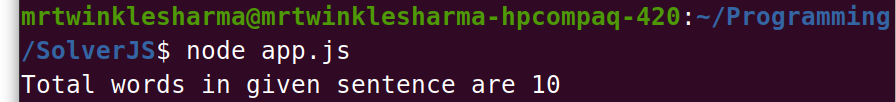Example 2: Here we are finding total permutations of a string, function permutation() receives a string and returns an array containing all of the permutations of that given string.

## app.js

 `const solverjs = require(``'solverjs'``);`` ` `const anotherString = ``"GFG"``;``const totalPermutations = solverjs.permutation(anotherString);``console.log(`Total permutations of ``'GFG'` `are \${totalPermutations}`);`

Output: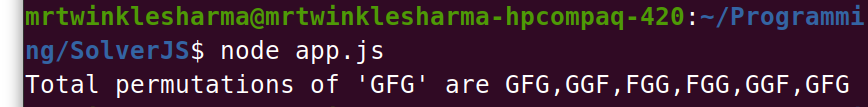6. Other Utilities: Some extra logics, which are used frequently like, these are the two examples in 1st one we are finding the day on a given date, must be in “dd/mm/yyyy” format and in 2nd one we are calculating age or can say the difference between the current date and given date.

Example 1: Here we are calculating the day on the given data, dateToDay() this function receives a date as an argument and returns the day occurring on that date.

## app.js

 `const solverjs = require(``'solverjs'``);`` ` `const date = ``"28/07/2021"``;``const day  = solverjs.dateToDay(date);``console.log(`There is \${day} on \${date}`);`

Output: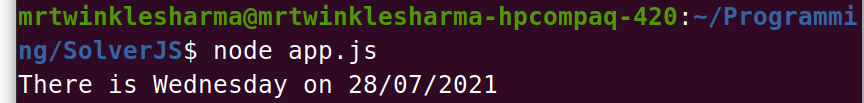Example 2: Here we are calculating the age according to the given date of birth. function dobToAge() receives the date in “dd/mm/yyyy” format, as an argument. After it calculates and returns the difference between the current date and the given one.

## app.js

 `const solverjs = require(``'solverjs'``);`` ` `const dateOfBirth = ``"25/01/2001"``;``const calculatedAge = solverjs.dobToAge(dateOfBirth);``console.log(`The age according to DOB \${dateOfBirth} is \${calculatedAge}`);`

Output: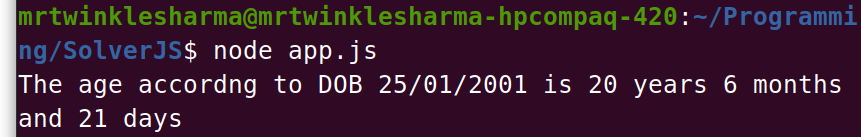Not only these, but SolverJS contains much more methods than we have explained, you can check them all from their Package Docs.

My Personal Notes arrow_drop_up